Estimating and localizing the algebraic and total errors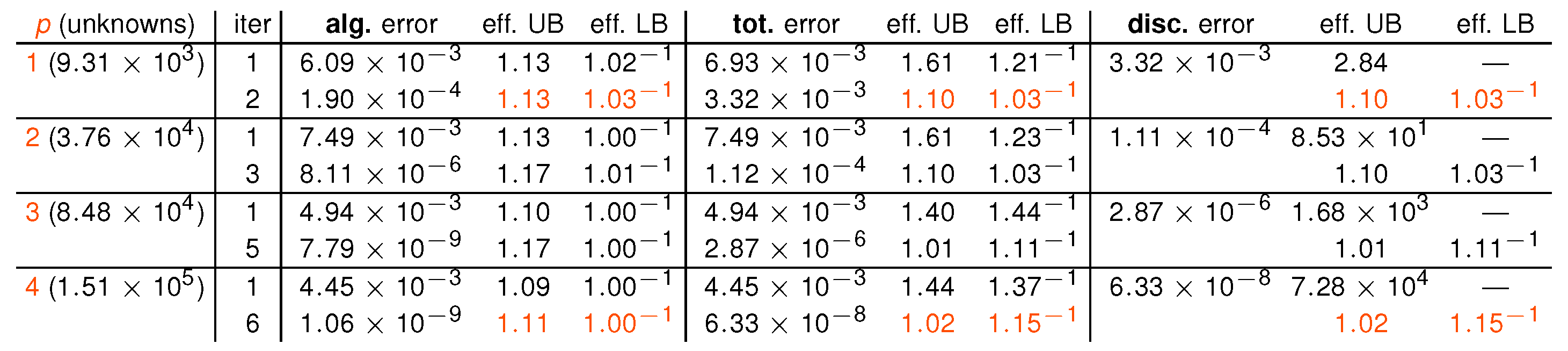Effectivity indices, smooth solution, geometric V-multigrid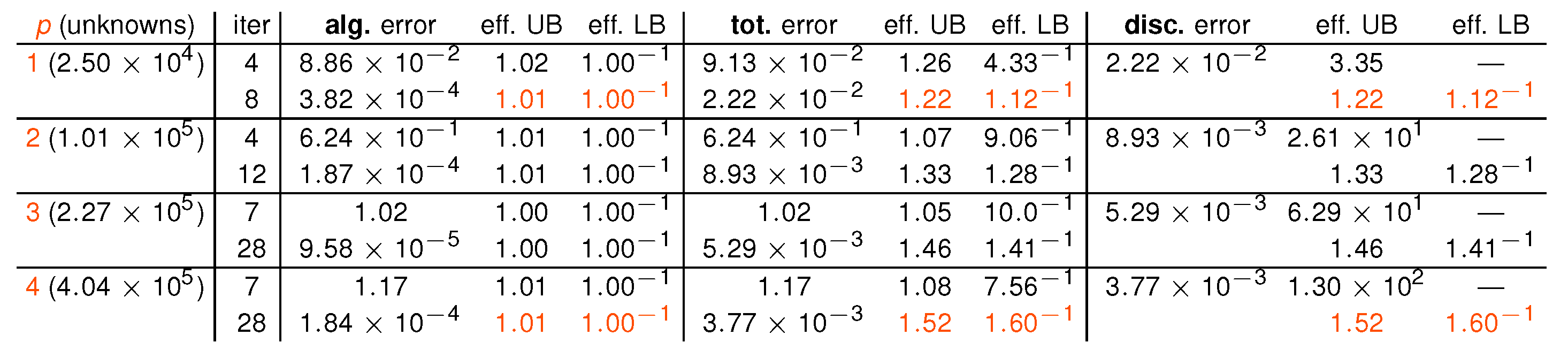Effectivity indices, singular solution, preconditioned conjugate gradients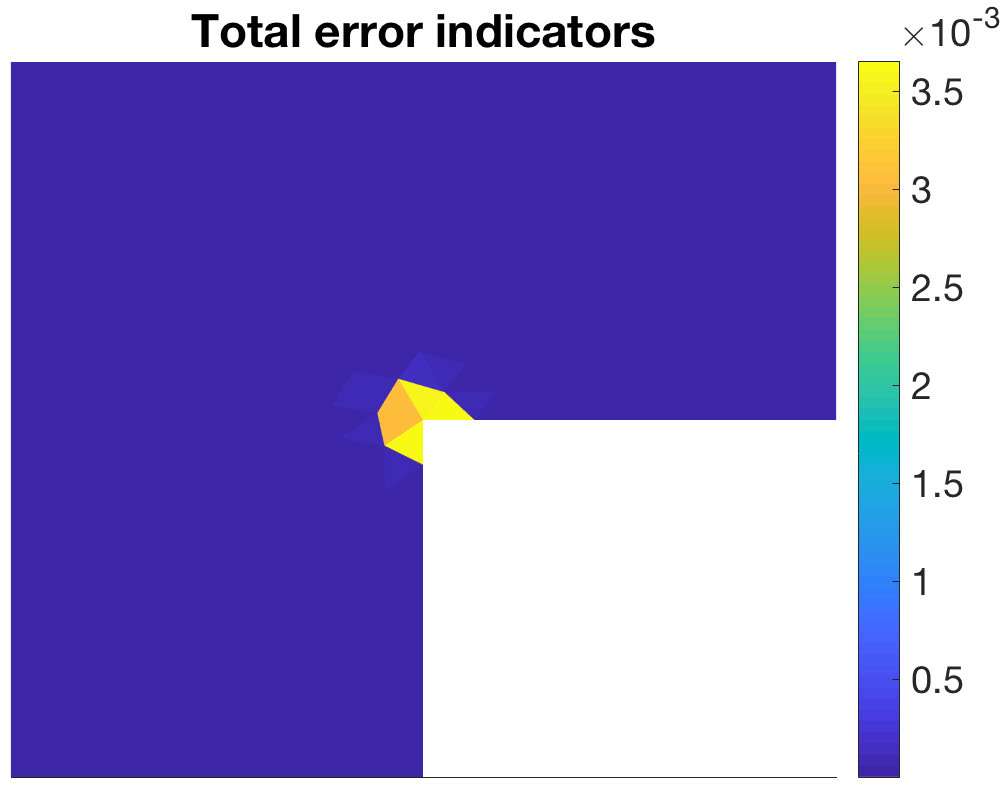Total estimator distribution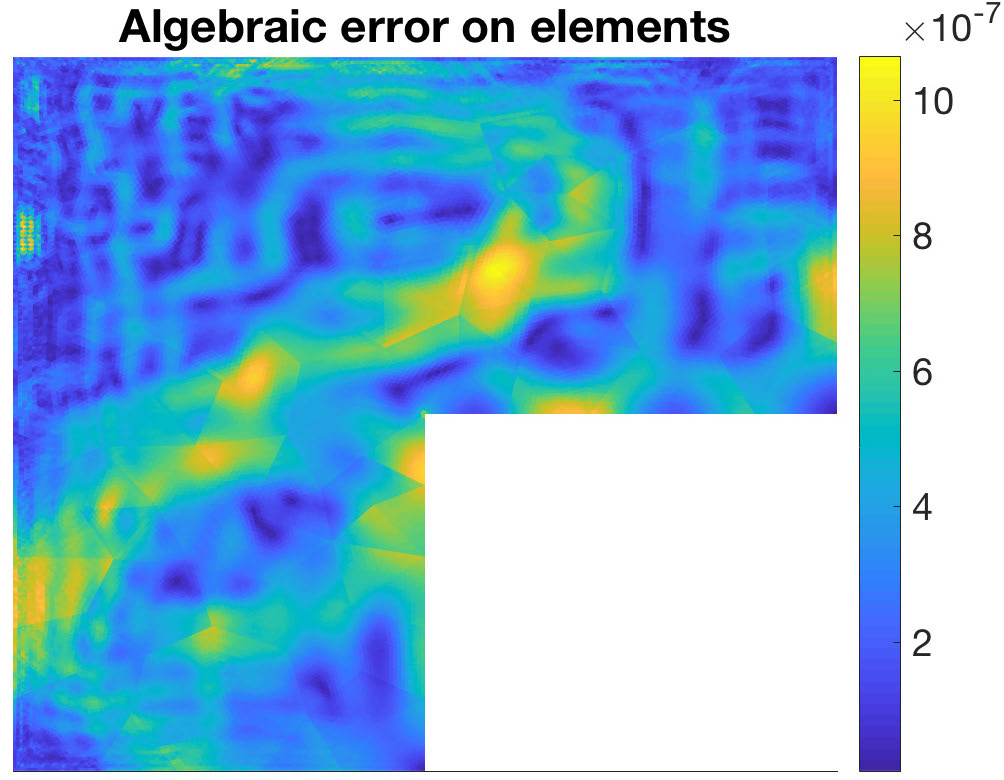Algebraic error distribution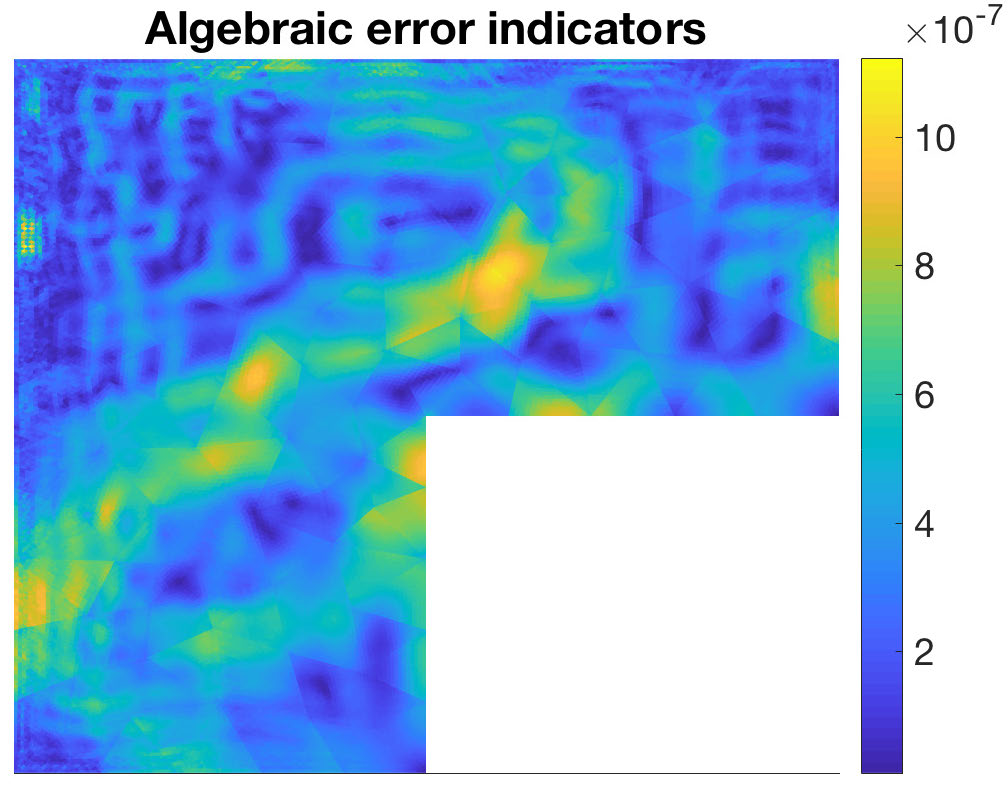Algebraic estimator distribution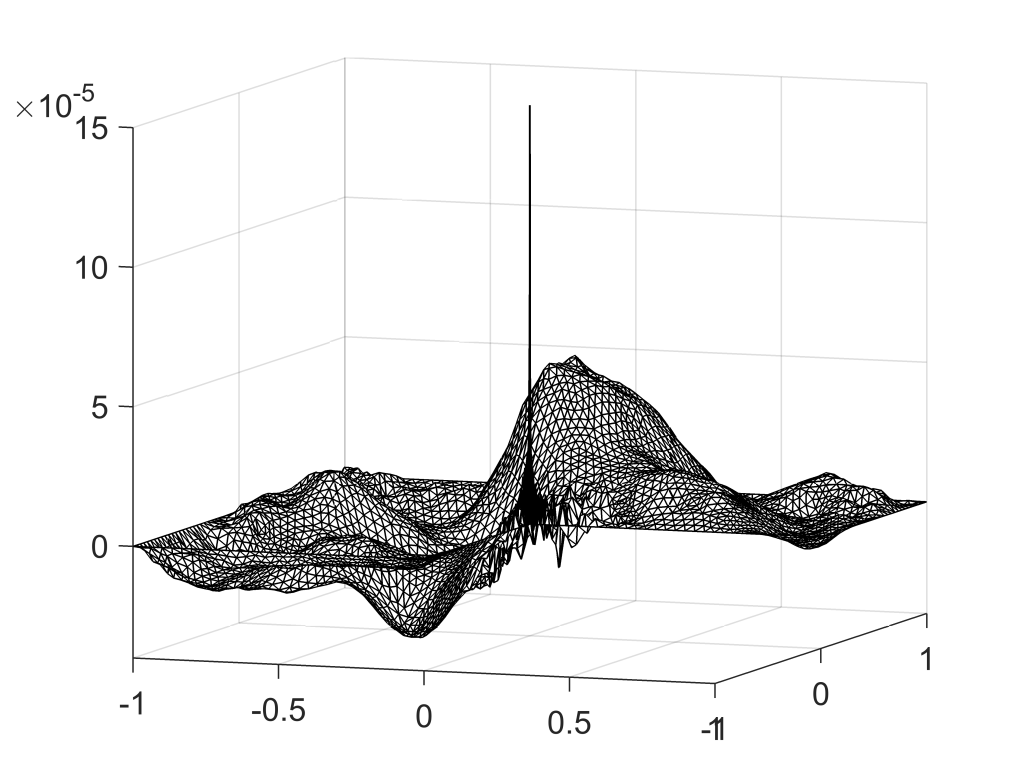Total misfit for a global stopping criterion: the shape is determined by algebraAlgebraic misfit for the global stopping criterionTotal misfit for a local stopping criterion: the shape is determined by discretization

Main results:

• total, algebraic, and discretization errors: guaranteed upper and lower bounds;
• valid for arbitrary iterative algebraic solver;
• estimating the local distribution of the errors over the computational domain;
• safe stopping criteria for iterative algebraic solvers: the algebraic error will lie below the discretization one;
• reliability and efficiency;
• recovering mass balance in any situation.

Details:

• via additional iterations: paper (preprint) with Jan Papež and Zdeněk Strakoš;
• via a multi-level approach: paper (preprint) with Jan Papež, Ulrich Rüde, and Barbara Wohlmuth;
• inexpensive multi-level construction: paper (preprint) with Jan Papež;
• presentation.